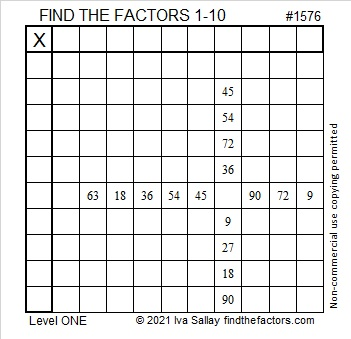# 1576 Why Do the Digits of Every Clue in This Puzzle Add up to Nine?

Contents

### Today’s Puzzle:

The digits of each clue in this puzzle add up to nine. Relatively few Find the Factors puzzles can make that claim. Why can this one make it?

Can you write all the numbers from 1 to 10 so that this puzzle will function like a multiplication table?### Factors of 1576:

1576 is divisible by a few powers of two, namely 1, 2, 4, and 8. Here is something for you to think about:

1576 is a whole number so it is divisible by 1.
6 is even so 1576 is divisible by 2.
7 is odd and 6 is not divisible by 4, so 1576 is divisible by 4.
5 is odd and 76 is not divisible by 8, so 1576 is divisible by 8.
1 is odd and 576 is divisible by 16, so 1576 is not divisible by 16.
(Each of those statements was also dependent on the statement before it.)

• 1576 is a composite number.
• Prime factorization: 1576 = 2 × 2 × 2 × 197, which can be written 1576 = 2³ × 197.
• 1576 has at least one exponent greater than 1 in its prime factorization so √1576 can be simplified. Taking the factor pair from the factor pair table below with the largest square number factor, we get √1576 = (√4)(√394) = 2√394.
• The exponents in the prime factorization are 3 and 1. Adding one to each exponent and multiplying we get (3 + 1)(1 + 1) = 4 × 2 = 8. Therefore 1576 has exactly 8 factors.
• The factors of 1576 are outlined with their factor pair partners in the graphic below.### More about the Number 1576:

1576 is the sum of two squares:
30² + 26² = 1576.

1576 is the hypotenuse of a Pythagorean triple:
224-1560-1576, calculated from 30² – 26², 2(30)(26), 30² + 26².
It is also 8 times (28-195-197).

1576 is also the difference of two squares in two different ways:
395² – 393² = 1576 and
199² – 195² = 1576.

This site uses Akismet to reduce spam. Learn how your comment data is processed.